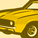# App Development

Announcements
April 22, 2PM EST: Learn about GeoOperations in Qlik Sense SaaS READ MORE
cancel
Showing results for
Did you mean:Creator

## Error in expression: Nested aggregation not allowed

Hi,

Can anyone help me to understand how to aggregate this expression?

I am having a hard time to understand how the Aggr function works.

```Avg(
(Count({<[Retroactiveness IT00]={'On Time'},Act.={'ZA', 'ZC', 'ZE', 'ZD'}>}[Retroactiveness IT00])/Count({<Act.={'ZA', 'ZC', 'ZE', 'ZD'}>} [Retroactiveness IT00]))
+
(Count({<[Retroactiveness IT00]={'On Time'},Act.={'ZA', 'ZC', 'ZE', 'ZD'}>}[Retroactiveness IT00])/Count({<Act.={'ZA', 'ZC', 'ZE', 'ZD'}>} [Retroactiveness IT00]))
+
(Count({<[Retroactiveness IT00 (New)]={'On Time'},Act.={'ZB','ZF'}>}[Retroactiveness IT00 (New)])/Count({<Act.={'ZB','ZF'}>}[Retroactiveness IT00 (New)]))
+
(Count({<[Retroactiveness IT00]={'On Time'},Act.={'ZN', 'ZO', 'ZM'}>}[Retroactiveness IT00])/Count({<Act.={'ZN', 'ZO', 'ZM'}>} [Retroactiveness IT00])))```

Thanks a lot!

Labels (1)

1 Solution

Accepted SolutionsPartner

Hi,

In you formula you're getting four ratio Count(x)/Count(y) numbers, then summing them up and trying to apply avg on top...

If you technically want to get AVG value of 4 value you can use RangeAvg(exp1, exp2, exp3,exp4) function, so you'll get

```RangeAvg(
(Count({<[Retroactiveness IT00]={'On Time'},Act.={'ZA', 'ZC', 'ZE', 'ZD'}>}[Retroactiveness IT00])/Count({<Act.={'ZA', 'ZC', 'ZE', 'ZD'}>} [Retroactiveness IT00]))
,
(Count({<[Retroactiveness IT00]={'On Time'},Act.={'ZA', 'ZC', 'ZE', 'ZD'}>}[Retroactiveness IT00])/Count({<Act.={'ZA', 'ZC', 'ZE', 'ZD'}>} [Retroactiveness IT00]))
,
(Count({<[Retroactiveness IT00 (New)]={'On Time'},Act.={'ZB','ZF'}>}[Retroactiveness IT00 (New)])/Count({<Act.={'ZB','ZF'}>}[Retroactiveness IT00 (New)]))
,
(Count({<[Retroactiveness IT00]={'On Time'},Act.={'ZN', 'ZO', 'ZM'}>}[Retroactiveness IT00])/Count({<Act.={'ZN', 'ZO', 'ZM'}>} [Retroactiveness IT00]))
)```

However, you should be very careful with calculating AVG of ratios... it's a very tricky thing.

Hope this helps.

//AndreiPartner

Hi,

In you formula you're getting four ratio Count(x)/Count(y) numbers, then summing them up and trying to apply avg on top...

If you technically want to get AVG value of 4 value you can use RangeAvg(exp1, exp2, exp3,exp4) function, so you'll get

```RangeAvg(
(Count({<[Retroactiveness IT00]={'On Time'},Act.={'ZA', 'ZC', 'ZE', 'ZD'}>}[Retroactiveness IT00])/Count({<Act.={'ZA', 'ZC', 'ZE', 'ZD'}>} [Retroactiveness IT00]))
,
(Count({<[Retroactiveness IT00]={'On Time'},Act.={'ZA', 'ZC', 'ZE', 'ZD'}>}[Retroactiveness IT00])/Count({<Act.={'ZA', 'ZC', 'ZE', 'ZD'}>} [Retroactiveness IT00]))
,
(Count({<[Retroactiveness IT00 (New)]={'On Time'},Act.={'ZB','ZF'}>}[Retroactiveness IT00 (New)])/Count({<Act.={'ZB','ZF'}>}[Retroactiveness IT00 (New)]))
,
(Count({<[Retroactiveness IT00]={'On Time'},Act.={'ZN', 'ZO', 'ZM'}>}[Retroactiveness IT00])/Count({<Act.={'ZN', 'ZO', 'ZM'}>} [Retroactiveness IT00]))
)```

However, you should be very careful with calculating AVG of ratios... it's a very tricky thing.

Hope this helps.

//AndreiTags
Community Browser